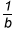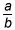Activities

••• Subject Area

• Math: Elementary Math: Fractions

• AuthorK-5

30 Minutes

• Device
•TI-Nspire™ CX/CX II
•TI-Nspire™ CX CAS/CX II CAS
• Software

TI-Nspire™
TI-Nspire™ CAS

Dividing a Whole Number by a Fraction

Activity Overview

This activity uses unit squares to illustrate the division of a whole number by a unit fraction.

This TI-Nspire™ lesson uses unit squares to illustrate the division of a whole number, c, by a unit fraction. Once this concept has been established, students build on this thinking to analyze representations of dividing the whole number, c, by the fraction. The activity also allows students to approach the task by considering the relationship between multiplication and division and identifying a missing factor.